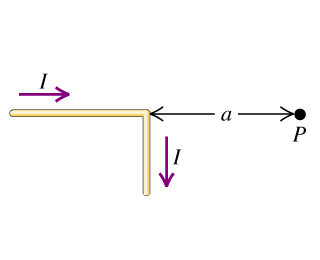# Problem: The wire shown in the figure (Figure 1) is infinitely long and carries a current.A. Calculate the magnitude of the magnetic field that this current produces at point P.B. Find the direction of the magnetic field that this current produces at point P.

###### FREE Expert Solution

From Biot-Savart Law:

$\overline{)\begin{array}{rcl}\mathbf{d}{\stackrel{\mathbf{⇀}}{\mathbf{B}}}_{\mathbf{h}\mathbf{o}\mathbf{r}\mathbf{i}\mathbf{z}\mathbf{o}\mathbf{n}\mathbf{t}\mathbf{a}\mathbf{l}}& {\mathbf{=}}& \mathbf{\left(}\frac{{\mathbf{\mu }}_{\mathbf{0}}}{\mathbf{4}\mathbf{\pi }}\mathbf{\right)}\mathbf{\left(}\frac{\mathbf{i}\mathbf{d}\stackrel{\mathbf{⇀}}{\mathbf{l}}\mathbf{×}\stackrel{\mathbf{^}}{\mathbf{r}}}{{\mathbf{r}}^{\mathbf{2}}}\mathbf{\right)}\\ & {\mathbf{=}}& \frac{{\mathbf{\mu }}_{\mathbf{0}}}{\mathbf{4}\mathbf{\pi }}\frac{\mathbf{i}\mathbf{d}\stackrel{\mathbf{⇀}}{\mathbf{l}}\mathbf{s}\mathbf{i}\mathbf{n}\mathbf{\theta }}{{\mathbf{r}}^{\mathbf{2}}}\end{array}}$

The magnetic field due to the infinite current-carrying wire is:

$\overline{)\stackrel{\mathbf{⇀}}{\mathbf{B}}{\mathbf{=}}\frac{{\mathbf{\mu }}_{\mathbf{0}}\mathbf{i}}{\mathbf{2}\mathbf{\pi }\mathbf{r}}}$

A.

For the horizontal wire, θ = 0°

Since sin(0°) = 0

dBhorizontal = 0###### Problem Details

The wire shown in the figure (Figure 1) is infinitely long and carries a current.A. Calculate the magnitude of the magnetic field that this current produces at point P.

B. Find the direction of the magnetic field that this current produces at point P.